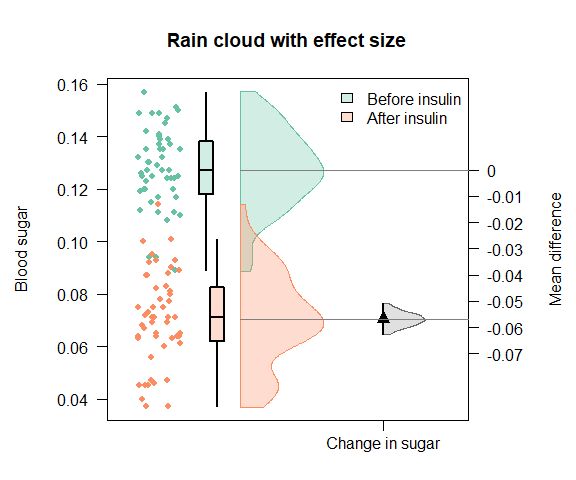# Introduction to Durga

Durga is an R package that aims to simplify the estimation and visualisation of one type of effect size: differences in group means. The package consists of two main functions and several auxiliary functions. The DurgaDiff function estimates raw or standardised mean differences, while DurgaPlot will create a plot for visualising both the original data and the effect size, and provides an extensive range of display options. The function DurgaBrackets can add confidence brackets to an existing Durga plot. Since Durga is implemented in base R, Durga plots can be positioned using the standard R plotting layout tools: par(mfrow = c(...)), layout() and split.screen().

This vignette shows some example Durga plots. Each plot is preceded by the code used to generate it, which can be used to learn how to create the plot.

## Effect size estimation

Before plotting your data, you must estimate the differences in group means by calling DurgaDiff. Your raw data should be in a data frame (or similar), with one observation per row - this is known as long format data. The data frame must contain a column for the measurement or value being analysed, and another for the group or treatment. In addition, paired or repeated measures data requires an ID column to match measurements for the same specimen/individual. For example, within the insulin data set, the value being measured - blood sugar level - is recorded in the column sugar (specified in the DurgaDiff call by passing the argument data.col = "sugar"); treatments are "before" and "after" in the treatment column (group.col = "treatment"); and the identity of the individual (rabbit) being tested is given in the id column (id.col = "id"). The blood sugar level of each rabbit was tested before and after treatment with insulin, so for each rabbit, there is a row with the before sugar level, and another with the after sugar level.

# Inspect the insulin data set
head(insulin[insulin$id < 4, ]) ## sugar treatment id experimenter_time time ## 1 0.111 before 1 ECN FEB 16 NA ## 2 0.139 before 2 ECN APR 22 NA ## 3 0.129 before 3 ECN APR 22 NA ## 53 0.045 after 1 ECN FEB 16 35 ## 54 0.075 after 2 ECN APR 22 45 ## 55 0.056 after 3 ECN APR 22 60 # Calculate differences in group means d <- DurgaDiff(insulin, data.col = "sugar", group.col = "treatment", id.col = "id") d ## Bootstrapped effect size ## sugar ~ treatment ## Groups: ## mean median sd se CI.lower CI.upper n ## after 0.07038462 0.071 0.01785250 0.002475697 0.06541445 0.07535478 52 ## before 0.12726923 0.127 0.01522397 0.002111185 0.12303085 0.13150761 52 ## Paired Mean difference (R = 1000, bootstrap CI method = bca): ## before - after: 0.0568846, 95% CI [0.0511754, 0.0624615] You may alternatively specify your data with a formula. Formulae allow a little more flexibility when specifying your model, but the end results are equivalent. The formula must be specified as <data variable> ~ <group variable>. For paired data, you must specify the id column using the id.col argument. # Use a formula to define the model DurgaDiff(sugar ~ treatment, insulin, id.col = "id") ## Bootstrapped effect size ## sugar ~ treatment ## Groups: ## mean median sd se CI.lower CI.upper n ## after 0.07038462 0.071 0.01785250 0.002475697 0.06541445 0.07535478 52 ## before 0.12726923 0.127 0.01522397 0.002111185 0.12303085 0.13150761 52 ## Paired Mean difference (R = 1000, bootstrap CI method = bca): ## before - after: 0.0568846, 95% CI [0.0512662, 0.0626584] ## Plotting The simplest plot to create simply uses all default values. This plot shows raw data points displayed as individual data points, the distribution of data points within each group shown as half violins, and effect size to the right (for a single group comparison) or beneath the group data (for multiple comparisons). The effect size is an estimate of the difference in means between two groups (or a similar statistic). It is shown with a symbol (a triangle by default) at the estimated value, and a thick line showing the confidence interval of the estimate. A filled half-violin shows the distribution of bootstrapped effect sizes, truncated at the top and bottom so it does not extend past the confidence interval. If the data are paired (aka repeated measures; id.col was specified in the DurgaDiff call), then pair lines are drawn to connect measurements for the same individual/specimen. DurgaPlot(d)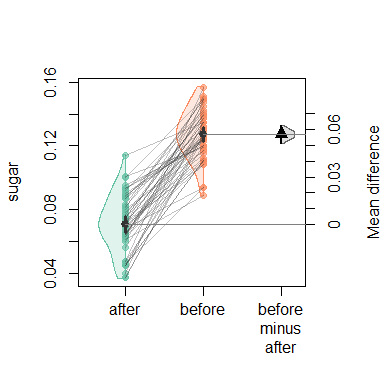### Plot components A DurgaPlot data visualisation is a combination of one or more visual components: data points; violin plots; bar charts; box plots; repeated measure paired lines; a mean or median indicator (central.tendency); and error bars (error.bars). Each component is controlled by a set of parameters to the DurgaPlot function. The names of the parameters indicate the component they apply to, so to adjust the violin plot component, read the documentation about all parameters with prefix violin. Effect size display is controlled by parameters with prefix ef.size. Parameters have sensible default values, so for example, if you display bar charts (bar = TRUE), violin plots and the central tendency indicator will be turned off. Obviously, you are able to override the defaults if you wish. Refer to help for the DurgaPlot function for full details on all arguments. # Show two plots in the same figure, and increase margins so they don't look crowded par(mfrow = c(1, 2), mar = c(5, 5, 4, 5) + 0.1) DurgaPlot(d, # Display box plots box = TRUE, # Don't display data points points = FALSE, # Don't display paired-value lines paired = FALSE, main = "Box plot") par(mar = c(5, 5, 4, 1) + 0.1) DurgaPlot(d, # Display bar charts bar = TRUE, # Position effect size below ef.size.position = "below", # No paired-value lines paired = FALSE, main = "Bar chart")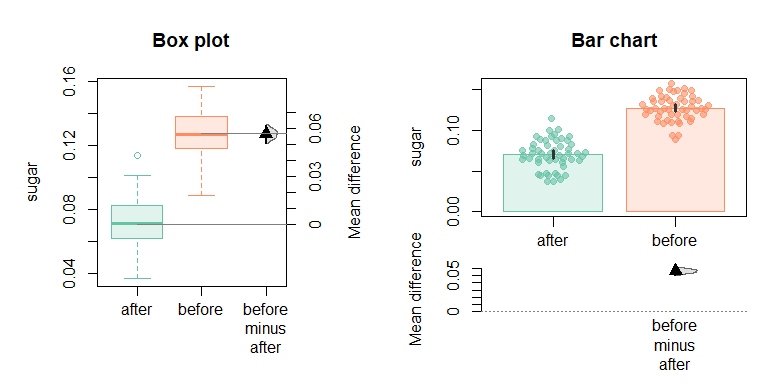### Group order and labels When plotted, the default display order for groups is their natural order, i.e. alphabetical order for character data, and numeric order for factors. You can, however, specify the display order with the groups parameter to either DurgaDiff or DurgaPlot. Not all groups in the data need be included in the analysis or plot; missing groups will be excluded from the analysis and/or plot. To assign display labels to the groups, use a named vector to list the groups. The names will be used to label the groups on the plot. In the damselfly data set, the groups are juvenile and adult. The example below demonstrates how to change the displayed group names as well as the display order of the groups. # No title on this plot, so reduce the top margin size par(mar = c(5, 4, 1, 1) + 0.1) # Immature males are yellow, mature are red. Display juveniles first, and label # with colour rather than developmental stage d <- DurgaDiff(mass ~ maturity, damselfly, na.rm = TRUE, groups = c("Yellow" = "juvenile", "Red" = "adult")) DurgaPlot(d, # Custom y-axis label left.ylab = "Body mass (mg)", # Specify the colours to (approximately) match the data group.colour = c("orange", "red"), box = TRUE, box.fill = FALSE)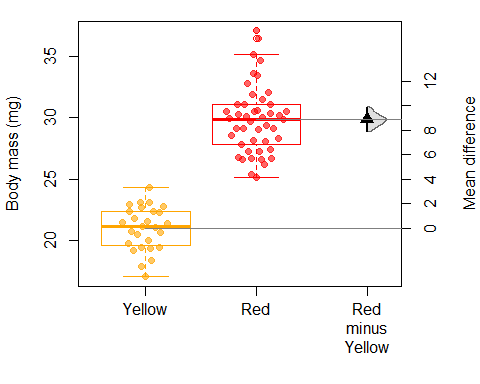### Colours Colours can be specified for each group (group.colour; demonstrated above), or separately for individual components. The master argument for each component type controls whether the component is displayed and optionally its colour. So, to display blue violins, specify violin = "blue". To use the default colour, specify violin = TRUE. Colours can be specified as the name of an RColorBrewer palette (e.g. group.colour = "Dark2"), or as one or more R colours. Colours for data points receive special treatment; points can be coloured individually or by group. If there are as many colours as data points, the points are coloured individually. Otherwise, colours are applied to groups (excess colours are ignored). The default point symbol (pch) is 19. The convenience function DurgaTransparent adds transparency to a colour, so DurgaTransparent("blue", 0.8) is a nearly-transparent blue colour. par(mfrow = c(1, 2), mar = c(11, 4, 3, 6) + 0.1) # Only show 2 groups even though there are 3 in the data d <- DurgaDiff(iris, data.col = "Sepal.Length", group.col = "Species", groups = c("versicolor", "setosa")) DurgaPlot(d, # Custom colours for points - the points are partially transparent points = c(DurgaTransparent("coral", .6), DurgaTransparent("darkturquoise", .8)), # Different colurs for violin (border) and violin fill violin = c("red", "blue"), violin.fill = c("#f8a8c840", "#a8c8f840"), main = "Data subset; Two groups") par(mar = c(5, 4, 3, 1) + 0.1) d <- DurgaDiff(iris, data.col = "Sepal.Length", group.col = "Species") # Define colours for the 3 species bgCols <- c(DurgaTransparent("red", 0.5), DurgaTransparent("blue", 0.5), DurgaTransparent("green", 0.5)) DurgaPlot(d, # Make all boxes the same colour box = "grey50", box.fill = "grey90", # Black for border/outline colour of points points = "black", # Additional data point parameters: small squares filled with colour for species points.params = list(pch = 22, cex = 0.8, bg = bgCols[iris$Species]),
main = "Coloured point fill")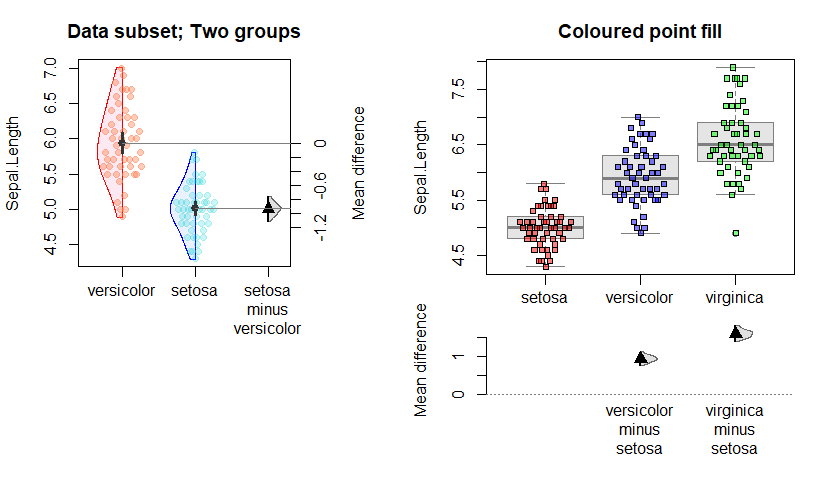### Central tendency and error bars

The central tendency indicator displays either the mean or median of each group (controlled by the central.tendency.type argument), and is shown by default when neither boxes nor bars are shown.

Error bars on each group may show: 95% confidence intervals of the mean; standard deviation; or standard error (specified by setting the error.bars.type parameter), and may be plotted regardless of what other components are shown.

par(mfrow = c(1, 2))
d <- DurgaDiff(iris, data.col = "Sepal.Length", group.col = "Species")

# Only show error bars (95% CI) and central tendency
DurgaPlot(d, error.bars.type = "CI",
# Custom colour for central tendency
central.tendency = rainbow(3),
points = FALSE, violin = FALSE, main = "Mean and 95% CI")

par(mar = c(5, 2.4, 4, 2) + 0.1)
DurgaPlot(d, bar = TRUE, error.bars.type = "SD", points = FALSE,
left.ylab = "", ef.size.label = "",
main = "Bar chart with std. deviation")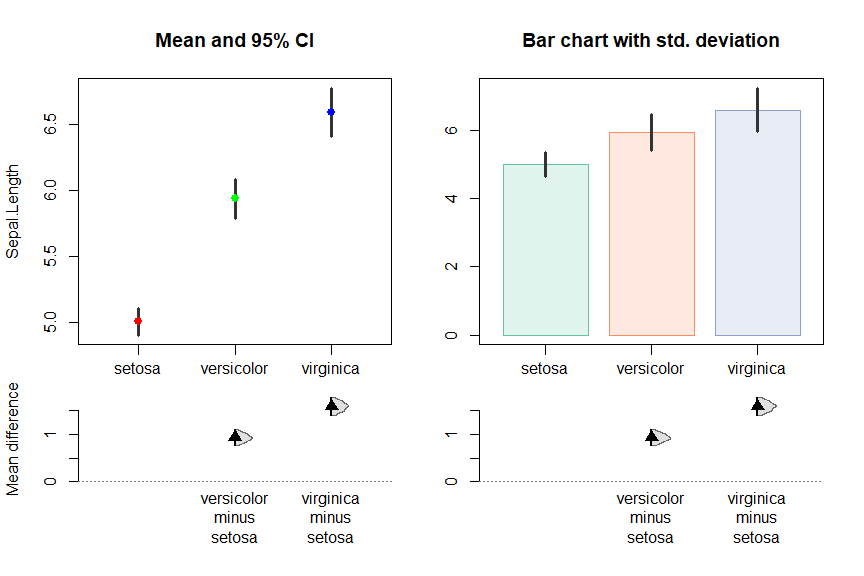### Multiple effect sizes

The default behaviour of DurgaDiff is to calculate differences between all pairs of groups; each difference is a contrast and has an estimated effect size with confidence interval. When plotted, each of multiple effect sizes is positioned below the first group in the contrast. This can cause layout problems when one group participates in multiple contrasts. For example, the iris dataset (available within standard R) contains three species: setosa, versicolor and virginica, hence three possible contrasts: virginica - versicolor, virginica - setosa and versicolor - setosa. If all three effect sizes are plotted, two of them (virginica - versicolor and virginica - setosa) will be positioned at the same horizontal location, resulting in superimposed text and an illegible graph (panel a) below)!

There are several ways around this problem:

1. display a subset of possible contrasts;
2. swap the order of groups in a contrast so there is at most one effect size underneath each group;
3. manually adjust effect size horizontal positions (achieved using the parameter ef.size.dx; exactly how to do this is left as an exercise for the reader);
4. use confidence brackets to depict effect sizes (described below).

There is no single best solution to this problem. The appropriate method depends on your data and the information that you wish to communicate.

To avoid this problem in default plots, the default behaviour of DurgaPlot is to assume that the first group is a control, and then show the differences between all subsequent groups and the control (method 1.). This behaviour prevents effect sizes from overwriting each other, but may exclude pertinant data from the plot (panel b) below).

To change the default contrast selection, specify the contrasts argument to either DurgaDiff or DurgaPlot. Contrasts are specified as "<group> - <group>, <group> - <group>", so to apply method 2. to the iris dataset, you could specify contrasts = c("virginica - setosa", "versicolor - setosa", "setosa - versicolor"). This differs from the default behaviour by also plotting the contrast "setosa - versicolor". Note that if the contrast "versicolor - setosa" were specified rather than "setosa - versicolor", the effect size would be shown at the same location as the "versicolor - setosa" effect size, hence failing to solve the problem.

par(mfrow = c(1, 2))

DurgaPlot(di, contrasts = "*", main = "Problematic effect sizes")
mtext("a)", cex = 1.5, col = "brown", font = 2, adj = -0.3, line = 1.4)

DurgaPlot(di, main = "Default effect sizes (subset)")
mtext("b)", cex = 1.5, col = "brown", font = 2, adj = -0.3, line = 1.4)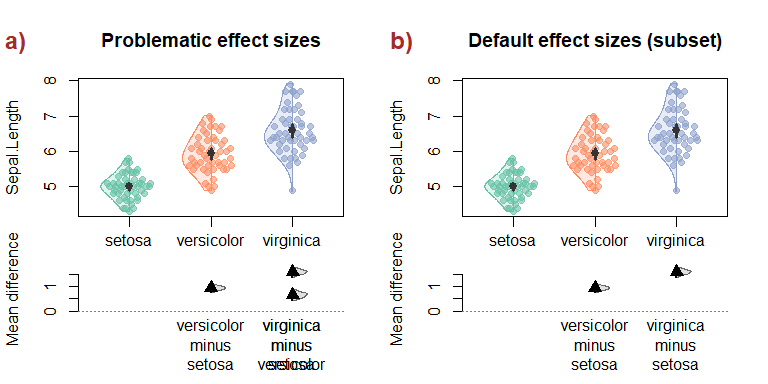### Confidence brackets

When there are too many group comparisons for the effect sizes to be clearly displayed, you can choose to display confidence brackets above the plot by calling DurgaBrackets after calling DurgaPlot. Confidence brackets communicate less information than the standard effect size visualisation, but may be a reasonable compromise for many group comparisons.

You must manually ensure there is sufficient space for the brackets in the plot (see DurgaBrackets for details). In the example below, to create space above the plot, we increase the top margin size and prevent the frame from being drawn around the plot (frame.plot = FALSE).

The default symbology for the brackets are light grey if the confidence interval includes 0. If the confidence interval does not include zero, text is black by default, and the default bracket line colour is the colour of the group with the higher central tendency. Arguments to DurgaBrackets can be used to override the defaults.

d <- DurgaDiff(petunia, 1, 2)
# Use the top margin for brackets
op <- par(mar = c(2, 4, 4, 1) + 0.1)

# Custom group colours
cols = rainbow(3)

# Save return value from DurgaPlot
p <- DurgaPlot(d,
# Don't draw effect size because we will draw brackets
ef.size = FALSE,
# Don't draw frame because brackets will appear in the upper margin
frame.plot = FALSE,
# Custom group colours
group.colour = cols)

# Customise colours by drawing brackets with the colour of the taller group.
# Get the taller group from each difference
tallerIdx <- sapply(d$group.differences, function(diff) { # If the group difference > 0, the first group is taller ifelse(diff$t0 > 0, diff$groupIndices, diff$groupIndices)
})

# Draw the brackets
DurgaBrackets(p, labels = "level CI", br.col = cols[tallerIdx])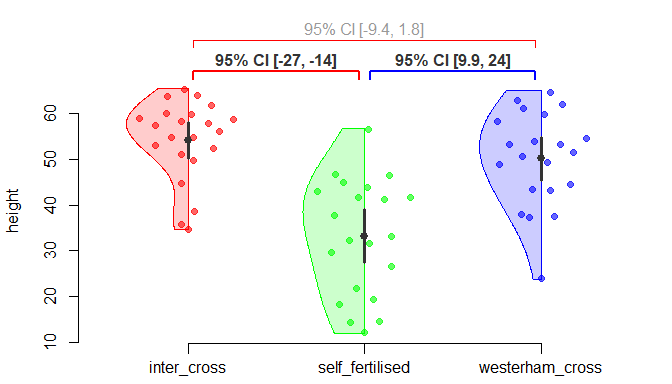### Layout

Each graphical group component (points, violin plot, box plot, bar chart, central tendency indicator, error bar and axis label) is positioned or centred horizontally on x-axis locations from 1 to n, where n is the number of groups. Group horizontal locations can be shifted with the group.dx parameter. For example, group.dx = 0.1 would shift all groups to the right by 1/10th of the distance between groups. Values are repeated as necessary, so group.dx = c(0.1, -0.1) will move each pair of groups closer together.

group.dx shifts all components equally, however, to provide fine-grained control, each component can be shifted independently. For example, to shift all violins left and all points right, specify violin.dx = -0.12, points.dx = 0.1.

par(mfrow = c(1, 2), mar = c(3, 4, 3, 1) + 0.1)

# Construct some data with multiple groups
set.seed(1) # Ensure we always get the exact same data set
multiGroupData <- data.frame(Measurement = unlist(lapply(c(10, 12, 8, 8.5, 9, 9.1),
function(m) rnorm(40, m))),
Group = rep(paste0("g", 1:6), each = 40),
Id = rep(1:6, 40))

DurgaPlot(d,
# Visually group each pair
group.dx = c(0.18, -0.18),
# Show box plots
box = TRUE,
# Make the boxes narrow
box.params = list(boxwex = 0.4),
# Don't display effect size or data points
ef.size = FALSE, points = FALSE,
xlim = c(0.8, 6.2), # Reduce white space on plot left and right
main = "Grouped into pairs")

DurgaPlot(d,
# Shift violins left
violin.dx = -0.12,
# Shift points right
points.dx = 0.1,
# Make points small and jittered
points.params = list(cex = 0.5), points.method = "jitter",
# No central tendency indicator
central.tendency = FALSE,
ef.size = FALSE, main = "Component shifts")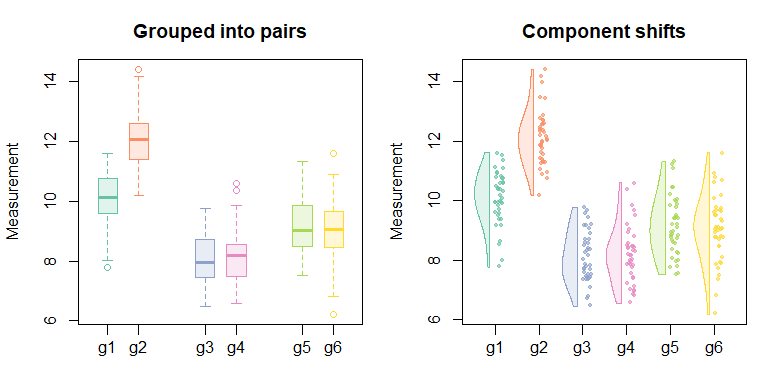### Labelling effect sizes

By default, effect sizes are “mean” (aka unstandardised), which means they are in the same units as the data. However, DurgaDiff can estimate standardised effect sizes - either Cohen’s d or Hedges’ g - which allow comparisons of effect sizes across data in different units.

You can override the y-axis labels to aid interpretation of effect sizes. DurgaPlot does not provide text labels for standardised effect sixes by default because effect size interpretation depends on context.

par(mar = c(5, 9, 3, 1) + 0.1)

# Define effect size labels and their positions on the y-axis
effectSizes <- c("No effect" = 0, "Large positive effect" = 0.8, "Huge positive effect" = 2)

# Give the groups friendly names
groups <- c("Self" = "self_fertilised", "Westerham" = "westerham_cross", "Inter" = "inter_cross")

# Calculate Cohen's d rather than unstandardised difference in means
d <- DurgaDiff(petunia, 1, 2, effect.type = "cohens", groups = groups)

DurgaPlot(d, violin = FALSE, main = "Cohen's d, labelled effect size",
points.method = "jitter",
# Use our ticks and labels instead of default
ef.size.ticks = effectSizes,
# Horizontal tick labels
ef.size.params = list(las = 1),
# Don't label the effect size y-axis because it won't fit with the long tick labels
ef.size.label = "")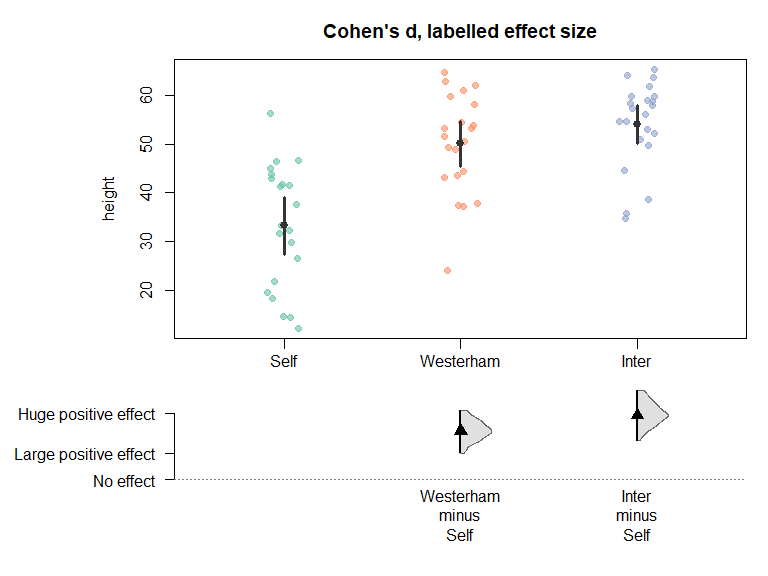### Custom statistics

Durga provides a fixed set of effect type statistics that can be used, however sometimes a different statistic is required. For example, we may wish to compare measurements of some organ or structure to know whether they have increased or decreased in size, and by how much, in which case we may decide to use log2 ratio as our statistic. log2 ratio has a straightforward interpretation: 0 means no difference, 1 means double the size, 2 means four-times the size, -1 means half the size, and so on.

# Define a function that calculates the statistic for two vectors
log2Ratio <- function(x1, x2) log2(mean(x2) / mean(x1))

# Generate some random data
set.seed(1)
data <- data.frame(species = rep(c("Sp1", "Sp2"), each = 10),
volume = c(rnorm(10, mean = 5, sd = 2), rnorm(10, mean = 10, sd = 2)))

# Apply log2Ratio as the effect type
d <- DurgaDiff(data, "volume", "species", effect.type = log2Ratio)

# Apply custom labels to describe the effect type, since it is not Sp2 - Sp1
d$group.differences[]$label.plot <- expression("log" ~ italic("Sp 2") * ":" * italic("Sp 1"))

# Plot, and set the y-axis label for effect size
DurgaPlot(d, ef.size.label = expression("log"~"ratio"))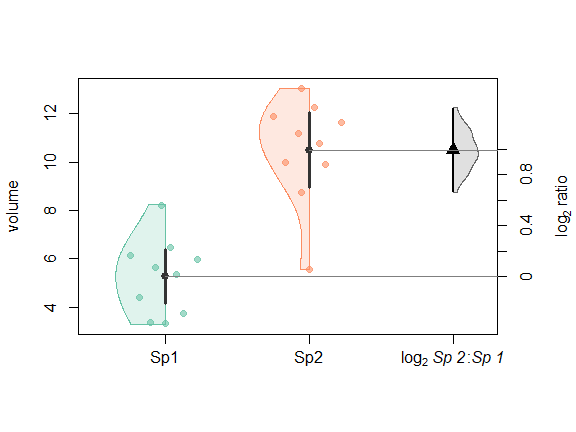# It is also possible to set the label to be used for printing to the console
d$group.differences[]$label.print <- "log2 Sp 2:Sp 1"
d
## Bootstrapped effect size
##   volume ~ species
## Groups:
##          mean    median       sd        se CI.lower  CI.upper  n
## Sp1  5.264406  5.513151 1.561172 0.4936859  4.14761  6.381201 10
## Sp2 10.497690 10.983745 2.139030 0.6764206  8.96752 12.027860 10
## Unpaired Custom effect type (R = 1000, bootstrap CI method = bca):
##   log2 Sp 2:Sp 1: 0.995729, 95% CI [0.669063, 1.32438]

## Examples

This example shows the use of custom group order and display names, contrasts between relevent pairs of groups rather than between all combinations of groups, and various custom plotting options.

par(mar = c(5, 4, 3, 1) + 0.1)

# Define display order and display pretty group names
groups = c("Control 1" = "g1", "Treatment 1" = "g2",
"Control 2" = "g3", "Treatment 2" = "g4",
"Control 3" = "g5", "Treatment 3" = "g6")
d <- DurgaDiff(multiGroupData, 1, 2, 3, groups = groups,
contrasts = "g2 - g1, g4 - g3, g6 - g5")
# Reduce text size
par(cex = 0.7)
DurgaPlot(d, box = TRUE,
# Group into pairs
group.dx = c(0.15, -0.15),
# Don't display points
points = FALSE,
# Adjust plot width to reduce left/right wasted space
xlim = c(1, 6),
# Don't display pair lines
paired = FALSE,
# Hide the boxplot median line (medcol = NA) and make the boxes narrow (boxwex = 0.4)
box.params = list(medcol = NA, boxwex = 0.4),
# Display mean and error bar
central.tendency = "#8060b0a0",      # Display and colour central tendency
central.tendency.symbol = "segment",
central.tendency.width = 0.07,
error.bars = "#8060b0a0",            # Same colour for error bars
# Don't display the effect size violin
ef.size.violin = FALSE,
main = "Group offsets"
)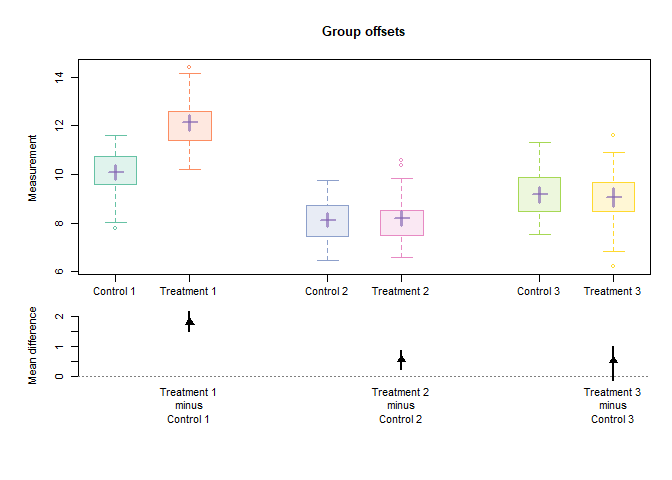Any unknown parameters to DurgaPlot are passed on to the plot function, which allows some control over additional aspects of the plot. The labels underneath effect sizes can be controlled by passing a named vector for the DurgaPlot contrasts parameter.

# Add in some fake sex data to the insulin data set
data <- cbind(insulin, Sex = sample(c("Male", "Female"), nrow(insulin), replace = TRUE))
# Thin the data so that individual symbols are visible.
# Obviously these data manipulations are for plot demonstration purposes only
data <- data[data$id %in% 1:15, ] d <- DurgaDiff(data, "sugar", "treatment", "id", groups = c("Before insulin" = "before", "After insulin" = "after"), na.rm = TRUE) par(mar = c(2, 4, 4, 1)) # Use different colours to show sex cols <- RColorBrewer::brewer.pal(3, "Set1") DurgaPlot(d, # Don't draw containing box frame.plot = FALSE, # Relabel the contrasts contrasts = c("Blood sugar change" = "after - before"), left.ylab = "Blood sugar level", # Customise the violins violin.shape = c("left", "right"), violin.dx = c(-0.055, 0.055), violin.width = 0.3, # Customise the points to display sex points = "black", points.params = list(bg = ifelse(data$Sex == "Female", cols, cols),
pch = ifelse(data$Sex == "Female", 21, 24)), # Customise the effect size ef.size.pch = 19, ef.size.violin = "blue", ef.size.violin.shape = "full", # Make mean lines match group colour ef.size.line.col = RColorBrewer::brewer.pal(3, "Set2")[2:1], ef.size.line.lty = 3, central.tendency = FALSE, main = "Effects of insulin on blood sugar") # Add a legend legend("topright", c("Female", "Male"), pch = c(21, 24), pt.bg = cols)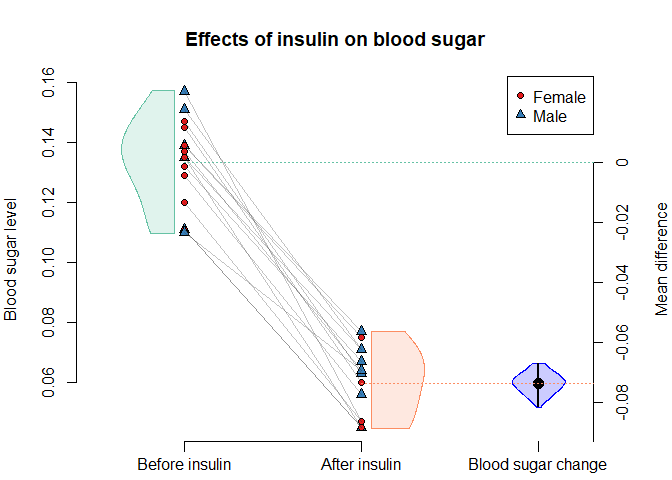Below is an alternative representation for the insulin dataset, showing overlayed rain cloud plots. Positioning is achieved by manually adjusting the relative positions of the various components, using the appropriate *.dx parameters. d <- DurgaDiff(insulin, "sugar", "treatment", "id", groups = c("Before insulin" = "before", "After insulin" = "after"), na.rm = TRUE) # Change effect size tick label d$group.differences[]\$label.plot <- "Change in sugar"

# Horizontal axis tick labels, move y-axis labels outwards to make space for the
# horizontal ticks, and ensure the margins are large enough to show them
par(las = 1, mgp = c(4, 1, 0), mar = c(3, 5.5, 4, 5.5) + 0.1)

cols <- RColorBrewer::brewer.pal(3, "Set2")
colsTr <- DurgaTransparent(cols, 0.7)
DurgaPlot(d, paired = FALSE,
# Move 2nd group to be at the same position as 1st
group.dx = c(0.2, -0.8),
# X-axis ticks are meaningless since both groups are at the same location
x.axis = FALSE,
# Adjust plot width and spacing
xlim = c(0.7, 2.1),
violin = TRUE, violin.shape = "right", violin.fill = colsTr,
# No need to specify violin position because violin.dx defaults to group.dx
# Bumpy violins
# Box plots are black outlines
box = "black", box.dx = c(0.06, -0.895), box.fill = colsTr,
# Don't show outliers
box.outline = FALSE,
# Thin boxes, solid thick lines
box.params = list(boxwex = 0.06, lty = 1, lwd = 2,
# normal line weight for median line
medlwd = 2,
# no end of whisker line
staplecol = NA),
# Points as solid colours, slightly smaller than default
points = cols, points.params = list(pch = 16, cex = 0.8),
points.method = "jitter", points.dx = c(-0.14, -1.14), points.spread = 0.09,
# Move effect size left to near where 2nd group would have been
ef.size.dx = -1.2,
left.ylab = "Blood sugar",
main = "Rain cloud with effect size"
)

legend("topright", c("Before insulin", "After insulin"), col = cols, fill = colsTr, bty = "n")